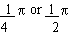Your browser does not support the IFRAME tag.Home MonkeyNotes Printable Notes Digital Library Study Guides Study Smart Parents Tips College Planning Test Prep Fun Zone Help / FAQ How to Cite New Title Request

 CHAPTER 4 : TRIGONOMETRIC IDENTITIES IDENTITY : When an equation contains one or more variables and it is valid for all replacement values of the variable for which both sides of the equation are well defined, then the equation is known as an identity. e.g. x2 + 2x + 1= (x + 1)2 But if an equation is true (or valid) for certain replacements of the variables concern, then it is known as "conditional equation". e.g. x2 - 3x + 2 = 0 In case of trigonometric equations i.e. equations involving trigonometric functions of unknown angles, if they are satisfied by all values of unknown angles in their domain of definitions; are known as identical equations or identities . While if they are satisfied only by particular values of unknown angles, they are called as conditional equations or simply equations. e.g. i) cos x sec x = 1 is an identity since for all values of x it is defined. ii) sin x = 0 is a conditional equation as forit is not satisfied. We have already seen a number of trigonometric identities. We restate them. Index Trignometric Identities 4.1 Fundamental Identities 4.2 The addition formulas 4.3 The multiple-angle (Double & Half angle) formulas 4.4 Tangent Identities 4.5 Factorization & Defactorization Supplementary Problems Chapter 5
 Search: All Products Books Popular Music Classical Music Video DVD Toys & Games Electronics Software Tools & Hardware Outdoor Living Kitchen & Housewares Camera & Photo Cell Phones Keywords: